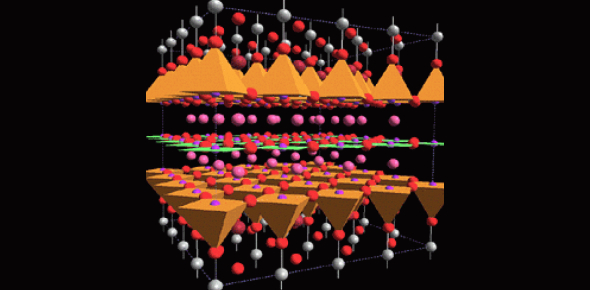# Trivia Facts On Matter: Quiz!

11 Questions | Total Attempts: 44SettingsEver wondered why is it essential to study matter? Have you ever given matter much thought? Scientists must study the properties of matter because everything is made up of matter. People are made up of matter as much as furniture is made up of matter. Each type of matter has different physical characteristics, and scientists must know these traits to make calculations. Whether you will pass this quiz is another matter. You can do it. Just go for it.

• 1.
Which of the following is not a mixture?
• A.

Solution

• B.

Homogeneous

• C.

Heterogeneous

• D.

Compound

• 2.
What is the study of the properties of matter?
• A.

Physics

• B.

Chemistry

• C.

Geology

• D.

Astronomy

• 3.
What is a combination of two or more atoms?
• A.

Molecule

• B.

Solution

• C.

Compound

• D.

Chemical bond

• 4.
What shows the elements in compounds and the ratio of atoms?
• A.

Physical property

• B.

Chemical bond

• C.

Chemical formula

• D.

Chemical property

• 5.
What is the matter?
• A.

Anything that takes up space

• B.

Anything that has mass

• C.

All of the above

• D.

A solid substance

• 6.
What can't be observed without changing it into another substance?
• A.

Chemical bond

• B.

Compound

• C.

Physical property

• D.

Chemical property

• 7.
You can see the different parts in any homogeneous mixture.
• A.

True

• B.

False

• 8.
What is a chemical formula?
• A.

Shows ratio of atoms

• B.

Shows elements in compounds

• C.

All of the above

• D.

The makeup of an atom

• 9.
How can you separate a mixture?
• A.

Magnetic attraction

• B.

Filtration

• C.

Distillation

• D.

All of the above

• 10.
H2O, NaCl, HCl (the written expressions) are all examples of what?
• A.

Substances

• B.

Chemical formulas

• C.

Chemical bonds

• D.

Compounds

• 11.
Which of these is not a physical property?
• A.

Melting point

• B.

Flammability

• C.

Density

• D.

Conductivity

Related TopicsBack to top